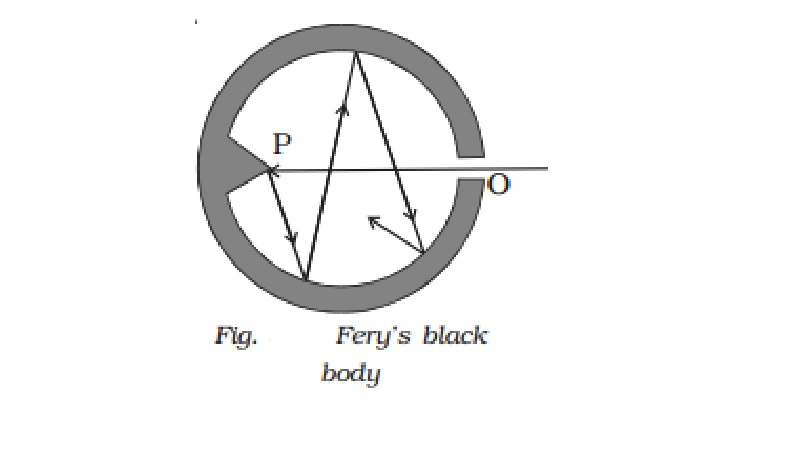Home | | Physics | | Physics | Perfect black body

# Perfect black body1 Fery?s black body 2.Prevost?s theory of heat exchanges 3 Kirchoff?s Law 4 Wien?s displacement law 5 Stefan?s law 6 Newton?s law of cooling

Perfect black body

A perfect black body is the one which absorbs completely heat radiations of all wavelengths which fall on it and emits heat radiations of all wavelengths when heated. Since a perfect black body neither reflects nor transmits any radiation, the absorptive power of a perfectly black body is unity.1 Fery?s black body

Fery?s black body consists of a double walled hollow sphere having a small opening O on one side and a conical projection P just opposite to it (Fig.). Its inner surface is coated with lamp black. Any radiation entering the body through the opening O suffers multiple reflections at its innerwall and about 97% of it is absorbed by lamp black at each reflection. Therefore, after a few reflections almost entire radiation is absorbed. The projection helps in avoiding any direct reflections which even otherwise is not possible because of the small opening O. When this body is placed in a bath at fixed temperature, the heat radiations come out of the hole. The opening O thus acts as a black body radiator.

2.Prevost?s theory of heat exchanges

Prevost applied the idea of thermal equilibrium to radiation. According to him the rate at which a body radiates or absorbs heat depends on the nature of its surface, its temperature and the temperature of the surroundings. The total amount of heat radiated by a body increases as its temperature rises. A body at a higher temperature radiates more heat energy to the surroundings than it receives from the surroundings. That is why we feel warm when we stand before the furnace.

Similarly a body at a lower temperature receives more heat energy than it loses to the surroundings. That is why we feel cold when we stand before an ice block.

Thus the rise or fall of temperature is due to the exchange of heat radiation. When the temperature of the body is the same as that of surroundings, the exchanges of heat do not stop. In such a case, the amount of heat energy radiated by the body is equal to the amount of heat energy absorbed by it.

A body will stop emitting radiation only when it is at absolute zero. (i.e) 0 K or ?273o C. At this temperature the kinetic energy of the molecule is zero.

Therefore, Prevost theory states that all bodies emit thermal radiation at all temperatures above absolute zero, irrespective of the nature of the surroundings.

3 Kirchoff?s Law

According to this law, the ratio of emissive power to the absorptive power corresponding to a particular wavelength and at a given temperature is always a constant for all bodies. This constant is equal to the emissive power of a perfectly black body at the same temperature and the same wavelength. Thus, if eλ is the emissive power of a body corresponding to a wavelength λ at any given temperature, aλ is the absorptive power of the body corresponding to the same wavelength at the same temperature and Eλ is the emissive power of a perfectly black body corresponding to the same wavelength and the same temperature, then according to Kirchoff?s law

eλ/ aλ = constant = Eλ

From the above equation it is evident that if aλ is large, then eλ will also be large (i.e) if a body absorbs radiation of certain wavelength strongly then it will also strongly emit the radiation of same wavelength. In other words, good absorbers of heat are good emitters also.

Applications of Kirchoff?s law

(i)The silvered surface of a thermos flask is a bad absorber as well as a bad radiator. Hence, ice inside the flask does not melt quickly and hot liquids inside the flask do not cool quickly.

(ii)                   Sodium vapours on heating, emit two bright yellow lines. These are called D1 and D2 lines of sodium. When continuous white light from carbon arc passes through sodium vapour at low temperature, the

continuous spectrum is absorbed at two places corresponding to the wavelengths of D1 and D2 lines and appear as dark lines. This is in accordance with Kirchoff?s law.

4 Wien?s displacement law

Wien?s displacement law states that the wavelength of the radiation corresponding to the maximum energy (λm) decreases as the temperature T of the body increases.

(i.e) λm T = b where b is called Wien?s constant. Its value is 2.898 ? 10-3 m K

5 Stefan?s law

Stefan?s law states that the total amount of heat energy radiated per second per unit area of a perfect black body is directly proportional to the fourth power of its absolute temperature.

(i.e) E α T4 or E = σT4

where σ is called the Stefan?s constant. Its value is 5.67 ? 10-8 W m-2 K-4.

It is also called Stefan - Boltzmann law, as Boltzmann gave a theoretical proof of the result given by Stefan.

6 Newton?s law of cooling

Newton?s law of cooling states that the rate of cooling of a body is directly proportional to the temperature difference between the body and the surroundings.

The law holds good only for a small difference of temperature. Loss of heat by radiation depends on the nature of the surface and the area of the exposed surface.

Study Material, Lecturing Notes, Assignment, Reference, Wiki description explanation, brief detail

Related Topics